## To teach computer programming to employees, many firms use on the job training. A human resources administrator wishes to review the perform

Question

To teach computer programming to employees, many firms use on the job training. A human resources administrator wishes to review the performance of trainees on the final test of the training. The mean of the test scores is 72 with a standard deviation of 5. The distribution of test scores is approximately normal. Find the z-score for a trainee, given a score of 82.

in progress 0
2 months 2021-08-03T09:24:24+00:00 1 Answers 2 views 0

The z-score for the trainee is of 2.

Step-by-step explanation:

Normal Probability Distribution

Problems of normal distributions can be solved using the z-score formula.

In a set with mean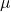and standard deviation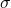, the z-score of a measure X is given by: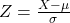The Z-score measures how many standard deviations the measure is from the mean. After finding the Z-score, we look at the z-score table and find the p-value associated with this z-score. This p-value is the probability that the value of the measure is smaller than X, that is, the percentile of X. Subtracting 1 by the p-value, we get the probability that the value of the measure is greater than X.

The mean of the test scores is 72 with a standard deviation of 5.

This means that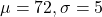Find the z-score for a trainee, given a score of 82.

This is Z when X = 82. So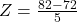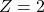The z-score for the trainee is of 2.